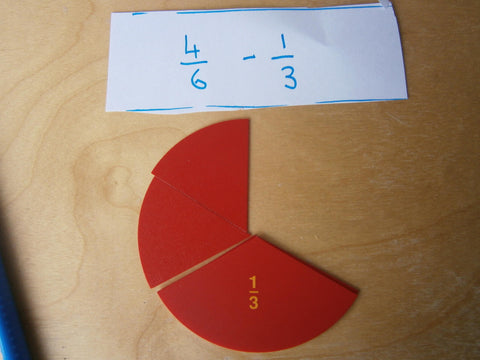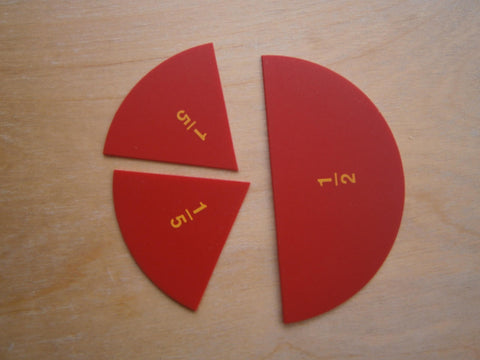# Subtracting fractions with Montessori

Subtracting FractionsThe lesson - SAME DENOMINATORS

Prior to this lesson students will need to

• have been introduced to adding fractions with the same denominator
1) Have some task cards with various subtraction equations of fractions on them
2) Have the montessori fraction pieces (the box of different pieces works best)
3) Place 7/8 in a frame. Check the students know you have put in eighths. Then remove two eighths Present the equation. Ask: 'How many eighths are left?' Write the answer.
4)  Have students record and if the presentation is given in a group explain to their partner what to do to solve an equation like this
5) Repeat using fraction equations and have students write answers into their bookThe lesson - UNLIKE DENOMINATORS

Prior to this lesson students would
• be very confident with equivalent fractions
1) Get out 4/6. Get out 1/3.  Write the equation 4/6 - 1/32) Discuss how the denominators are different. Ask if anyone knows how we can subtract these two numbers. Prompt students about equivalent fractions. If needed show how 1/3 covers 2/6 this must mean it is equivalent3)  Say the question could be rephrased as 4/6 subtract 2/6
5) Have students record and if the presentation is given in a group explain to their partner what to do to solve an equation like this
6) Use the subtraction of fraction tickets and do more questions.
____
The Lesson  - CHANGING BOTH DENOMINATORS
1) Record the question 1/2 - 2/5
2) Get out the fraction circles. Discuss how like other questions the child has done these fractions have different denominators. What have we done before in this case?
3) Ask if we can change the 1/2 into fifths (No) Can we change the 2/5 into halves (No)
4) We will have to change both of the fractions. Ask the students if they know a common multiple of 2 and 5 (10) Lets turn all of the fractions into this equation into tenths.5) 1/2 is how many tenths? (5) 2/5 are how many tenths (4)
6) 5/10 - 4/10 is 1/10
7) Have students record and if the presentation is given in a group explain to their partner what to do to solve an equation like this
8) Use the subtraction of fraction tickets to solve more questions.
Easy peasy ready to use subtraction of fraction tickets to use when teaching

Make sure to also check out

Teaching Improper fractions with Montessori

Teaching fractions with the Montessori stamp game

Interested in More Montessori Curriculum resources? Find them here

You can join my mailing list to get more montessori resources, notifications of new products and news here

## 1 comment

Hi, your post is very detailed and informative and your technique of teaching fractions is very effective. You are indeed in a different level. You held an activity and using some materials like montessori fractions pieces to further illustrate the whole meaning of fraction. Your student are very lucky to have you. Regarding adding and subtracting fractions, let me also share my idea on how to deal with fractions but I guess this is suitable to higher grades.

Adding and Subtracting fractions maybe difficult at first but keeping on practicing will make it easier in the long run. To add and subtract fractions successfully is to make the rules stick to your memory. So I have to mention again the rules here.

Rules are:
Same denominator:
Add both numerators then reduce. The result would be the final answer.
Different denominator (4 steps):
1. Multiply the numerator of first fraction to the denominator of second fraction. The result is the new numerator of first fraction.
2. Multiply the numerator of the second fraction to the denominator of first fraction. The result is the new numerator of second fraction.
3. Multiply both denominators. The result is the common denominator for two fractions.

To make it stick to your memory:
Rules for subtraction:
Same denominator:
Subtract second numerator from first then reduce. The result would be the final answer.
Different denominator (4 steps):
1. Multiply the numerator of first fraction to the denominator of second fraction. The result is the new numerator of first fraction.
2. Multiply the numerator of the second fraction to the denominator of first fraction. The result is the new numerator of second fraction.
3. Multiply both denominators. The result is the common denominator for two fractions.
4. Subtract new second numerator from first new numerator. The result is now the answer.

To make it stick to your memory:
Same numerator:
Subtract two fractions 50 times.
Different denominator: